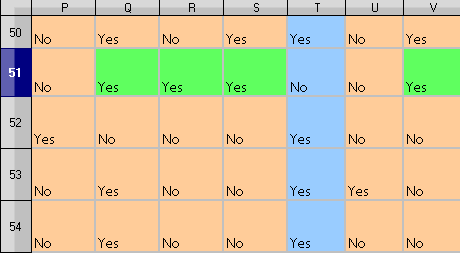# Help with formula

I have a test area(the real area is larger but contains the same values: YES, NO)

I want to count in row 50 all YES values but only when there is a YES value in the same column in row 51,

In the small test area shown above, I only want to count the YES in row 50 for column Q, S, V
(The YES in row 50 column T should not be counted)

I tried various combinations of COUNTIF and AND function but could not get the result needed.

I appreciate any hint

Hi ROSt52, I think this can be done with:

First question:
=SUMPRODUCT(\$A50:\$V50=“Yes”;\$A51:\$V51=“Yes”)

Second questions:
=SUMPRODUCT(\$A50:\$V50=“Yes”;COLUMN(\$A50:\$V50)<>COLUMN(\$T50))

@marosv - thanks for pointing to the SUMPRODUCT formula. This seems to be very powerful!

1. Helper row with formulas `=AND(P50="yes",P51="yes")` copied through column V, then `=COUNTIF(P[helperrow]:V[helperrow]="TRUE")` … can use `=(P50="yes")*(P51="yes")` in the helper row.
2. Replace all “yes” with “TRUE”, then `=sumproduct(P50:V50,P51:V51)`

@w_whalley - thanks for your hint. I will especially have a look the your No2 proposal